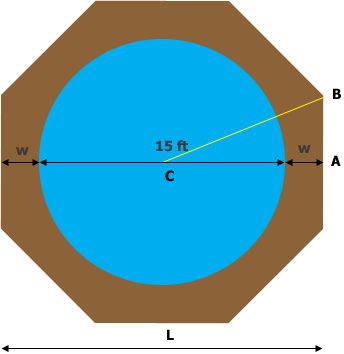SEARCH HOMEMath Central Quandaries & QueriesQuestion from Linda: My pool is 15 feet across and it is round. How do I measure to cut wood to build a sandbox around it? was thinking it will look like an octagon. ThanksHi Linda,

First you need to decide how wide to make the sandbox, the dimension $w$ feet in my diagram.Then $L = 15 + 2w$ feet and you can use the expression derived by Penny to show that the side length $s$ is given by $s = 0.4142 L.$

To cut the boards you will probably need to know the measure of the angle ABC. You can see this as the angle QPO in another of Penny's responses to one of our questions.

I hope this helps,

HarleyMath Central is supported by the University of Regina and The Pacific Institute for the Mathematical Sciences.